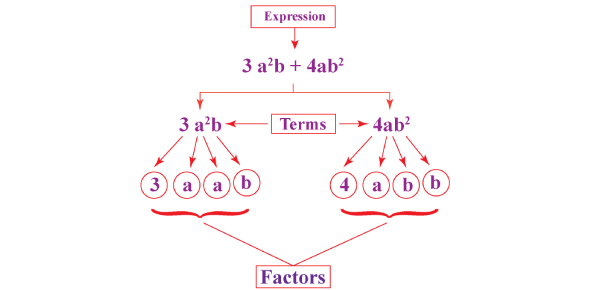# Factorization Of Algebraic Expressions Quiz Questions & Answers

Approved & Edited by ProProfs Editorial Team
At ProProfs Quizzes, our dedicated in-house team of experts takes pride in their work. With a sharp eye for detail, they meticulously review each quiz. This ensures that every quiz, taken by over 100 million users, meets our standards of accuracy, clarity, and engagement.
| Written by Tjkim
T
Tjkim
Community Contributor
Quizzes Created: 25 | Total Attempts: 57,155
Questions: 10 | Attempts: 10,371SettingsDo you know how to factorize algebraic expressions? Take this factorization of algebraic expressions quiz questions & answers to see how well you understand the concept. It is basically reducing the equations into a simpler form. To test your knowledge, take this quiz and check out your scores. If you want to practice or check your understanding, this quiz will help you. All the best! Go for it and ace it! Do share the quiz with those who wish to practice Algebra.

• 1.

### 9x + 24y

• A.

3(3x + 8y)

• B.

9x + 24y

• C.

X(9 + 24)

A. 3(3x + 8y)
Explanation
The given expression 9x + 24y is equivalent to the expression 3(3x + 8y) because when we distribute the 3 to both terms inside the parentheses, we get 3 multiplied by 3x which is 9x, and 3 multiplied by 8y which is 24y. Therefore, the two expressions are equal. The answer 3(3x + 8y) is the correct one.

Rate this question:

• 2.

### 7(y + 1) - x(y + 1)

• A.

7y + 7 - xy - x

• B.

(y + 1)(7x)

• C.

(y + 1)(7 - x)

C. (y + 1)(7 - x)
Explanation
The given expression can be simplified by distributing the terms. First, distribute 7 to both y and 1, which gives 7y + 7. Then, distribute -x to both y and 1, which gives -xy - x. Combining these terms, we get 7y + 7 - xy - x. This matches the expression (y + 1)(7 - x), so the answer is (y + 1)(7 - x).

Rate this question:

• 3.

### 5(x + 2) + y(x + 2)

• A.

(x + 2)(5 + y)

• B.

5x + 10 + xy + 2y

• C.

(2x)(5 + y)

A. (x + 2)(5 + y)
Explanation
The given expression can be simplified by distributing the terms inside the parentheses. By doing so, we get 5x + 10 + xy + 2y. This matches the expression (x + 2)(5 + y), so it is the correct answer.

Rate this question:

• 4.

### 20x - 10

• A.

10(2x - 1)

• B.

2(10x - 5)

• C.

10(x - 1)

A. 10(2x - 1)
Explanation
The given expression 20x - 10 can be factored out as 10(2x - 1). This is the correct answer because when we distribute 10 to the terms inside the parentheses, we get 20x - 10, which matches the original expression.

Rate this question:

• 5.

### 12mn + 18mp

• A.

6m(2n + 3p)

• B.

12(mn + 18mp)

• C.

2(6mn + 9mp)

A. 6m(2n + 3p)
Explanation
The given expression, 12mn + 18mp, can be factored out by taking out the common factors of 6m. This results in 6m(2n + 3p).

Rate this question:

• 6.

### X(x - 1) + 5(x - 1)

• A.

(x - 1)(5 -x)

• B.

(x - 1)(5x)

• C.

(x - 1)(x + 5)

C. (x - 1)(x + 5)
Explanation
The given expression can be simplified by factoring out the common factor of (x - 1) from both terms. This results in (x - 1) multiplied by (x + 5), which is the correct answer.

Rate this question:

• 7.

### 6a - 4b + 8c

• A.

2(3a - 2b + 4c)

• B.

A(6a - 4 + 8c)

• C.

4(2a - b + 2c)

A. 2(3a - 2b + 4c)
Explanation
The given expression 6a - 4b + 8c can be simplified by factoring out the common terms from each term. The expression 2(3a - 2b + 4c) is the correct answer because it represents the simplified form of the given expression. By distributing the 2 to each term inside the parentheses, we get 6a - 4b + 8c, which matches the original expression.

Rate this question:

• 8.

### 18x - 12y - 30z

• A.

6x(3x - 2y - 5z)

• B.

2(9x - 6y - 15z)

• C.

18(x - 12 - 30z)

A. 6x(3x - 2y - 5z)
Explanation
The given expression, 18x - 12y - 30z, can be factored out by finding the greatest common factor (GCF) of the coefficients. The GCF of 18, 12, and 30 is 6. Factoring out 6 from each term gives us 6x(3x - 2y - 5z). Therefore, the correct answer is 6x(3x - 2y - 5z).

Rate this question:

• 9.

### Factorize: x²+4x

• A.

X(x+4)

• B.

4x(x+1)

• C.

X(x+4)

• D.

None of the above

A. X(x+4)
Explanation
The given expression can be factorized as x(x+4). This is because we can factor out the common factor of x from both terms, leaving us with x multiplied by (x+4).

Rate this question:

• 10.

### Factorize: 10xy - 25yz

• A.

2xy - 5yz

• B.

5xy( 2 - 5z)

• C.

5y(2x - 5z)

• D.

10x - 25zBack to top